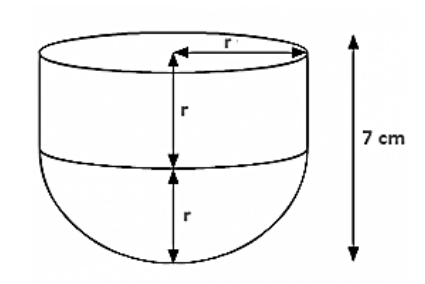# A cylinder of same height and radius is placed on top of a hemisphere.Question:

A cylinder of same height and radius is placed on top of a hemisphere. Find the curved surface area of the shape if the length of the shape is 7cm.

Solution:

Given length of the shape = 7cm

But length = r + r

2r = 7cm

r = 3.5 cm

Also; h = rTotal surface area of shape $=2 \pi r h+2 \pi r^{2}$

$=2 \pi r r+2 \pi r^{2}$

$=2 \pi r^{2}+2 \pi r^{2}$

$=4 \times 22 / 7 \times(3.5)^{2}=154 \mathrm{~cm}^{2}$